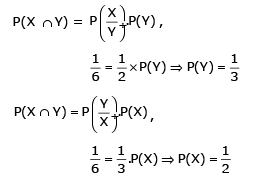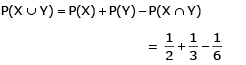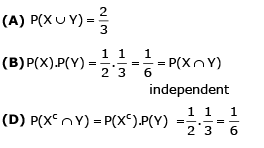Courses

# Test: Previous Year Questions - Probability (Competition Level)

## 44 Questions MCQ Test Mathematics (Maths) Class 12 | Test: Previous Year Questions - Probability (Competition Level)

Description
This mock test of Test: Previous Year Questions - Probability (Competition Level) for JEE helps you for every JEE entrance exam. This contains 44 Multiple Choice Questions for JEE Test: Previous Year Questions - Probability (Competition Level) (mcq) to study with solutions a complete question bank. The solved questions answers in this Test: Previous Year Questions - Probability (Competition Level) quiz give you a good mix of easy questions and tough questions. JEE students definitely take this Test: Previous Year Questions - Probability (Competition Level) exercise for a better result in the exam. You can find other Test: Previous Year Questions - Probability (Competition Level) extra questions, long questions & short questions for JEE on EduRev as well by searching above.
QUESTION: 1

### If the probability of solving a problem by three students are 1/2, 2/3 and 1/4 then probability that the problem will be solved- [AIEEE 2002]

Solution: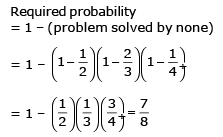QUESTION: 2

###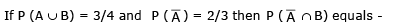[AIEEE 2002]

Solution: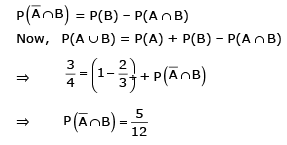QUESTION: 3

### A pair of dice is thrown. If 5 appears on at least one of the dice, then the probability that the sum is 10 or greater, is -[AIEEE 2002]

Solution:

Let A be the event that 5 appears on at least one of dice let B be the event that sum is 10 or greater.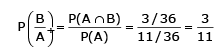QUESTION: 4

Events A, B, C are mutually exclusive events such that P(A)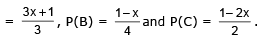The set of possible values of x are in the interval-

Solution: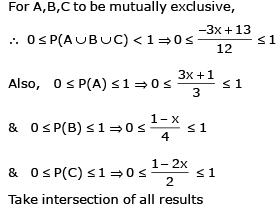QUESTION: 5

Five horses are in a race. Mr. A selects two of the horses at random and bets on them. The probability that Mr. A selected the winning horse is-

[AIEEE 2003]

Solution:

Let H1H2H3H4 B be the horses in which B is the winning horse.
Required probability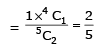QUESTION: 6

Probability of occurrence of an event lies between

Solution:
QUESTION: 7

A random variable X has the probabil ity distribution :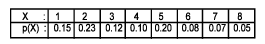For the events E = {X is a prime number} and F = {X < 4}, the probability P(E ∪ F) is

[AIEEE 2004]

Solution:

P (E) = P (2 or 3 or 5 or 7) = 0.23 + 0.12 + 0.20 + 0.07
= 0.6
P (F) = P (1 or 2 or 3)
= 0.15 + 0.23 + 0.12
= 0.50
P (E ⋂ F) = P (2 OR 3)=0.23+0.12=0.35
P (E ⋂ F) = P (E) + P (F) - P (E U F)
0.35 = 0.62 + 0.50 - P (E U F)
= 0.77

QUESTION: 8

The mean and the variance of binominal distribution are 4 and 2 respectively.Then the probability of 2 successes is-

[AIEEE 2004]

Solution:

mean =4
np=4 and variance =2
npq=2
⇒4q=2
⇒q=1/2
∴p=1−q
= 1−1/2 = 1/2 also n=8 probability of 2 successes
p(x=2)=8C2p2q6
=[8!/(2!×6!)]×(1/2)2×(1/2)^6
=28×1/2(8)= 28/256

QUESTION: 9

Three houses are available in a locality. Three persons apply for the houses. Each applies for one house without consulting others. The probability that all the three apply for the same house is

-[AIEEE-2005]

Solution:

Any person can apply for any house.
Required probability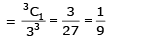QUESTION: 10

Let A and B be two events such that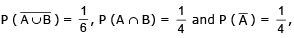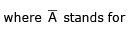complement of event A. Then events A and B are -

[AIEEE-2005]

Solution: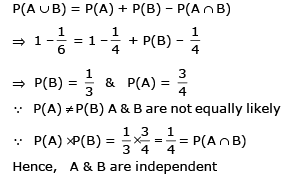QUESTION: 11

A pair of fair dice is thrown independently three times. The probability of getting a score of exactly 9 twice is-

[AIEEE 2007]

Solution:

Probability of getting a score of 9 twice is Required probability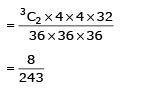QUESTION: 12

Two aeroplanes I and II bomb a target in succession. The probabilities of I and II scoring a hi t correctly are 0.3 and 0.2, respectively. The second plane will bomb only if the first misses the target. The probability that the target is hit by the second plane is-

[AIEEE 2007]

Solution:

Required probability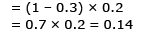QUESTION: 13

A die is thrown. Let A be the event that the number obtained is greater than 3. Let B be the event that the number obtained is less than 5. Then  P (A ∪ B) is

[AIEEE 2008]

Solution: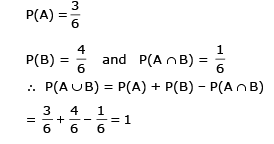QUESTION: 14

It is given that the events A and B are such that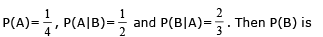[AIEEE 2008]

Solution: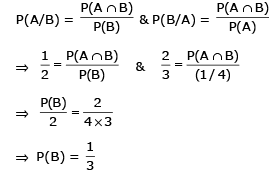QUESTION: 15

In a binomial distribution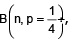f the probability of at least one success is greater than or equal to 9/10, then n is greater than :

[AIEEE 2009]

Solution: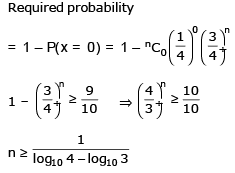QUESTION: 16

One ticket is selected at random from 50 tickets numbered 00, 01, 02, ......., 49. Then the probability that the sum of the digits on the selected ticket is 8, given that the product of these digits is zero, equals :

[AIEEE 2009]

Solution:

Let A be the event that sum of digits on selected ticket is 8 let B be the event that product is zero.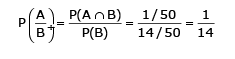QUESTION: 17

An urn contains nine balls of which three are red, four are blue and two are green. Three balls are drawn at random without replacement from the urn. The probability that the three balls have different colours is

[AIEEE 2010]

Solution:

Required probability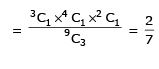QUESTION: 18

Four numbers are chosen at random (without replacement) from the set {1, 2, 3, ....., 20}.

Statement – 1: The probability that the chosen numbers when arranged in some order will form an AP is1/85.

Statement – 2: If the four chosen numbers form an AP, then the set of all possible values of common diference is {±1, ±2, ±3, ±4, ±5}

[AIEEE 2010]

Solution: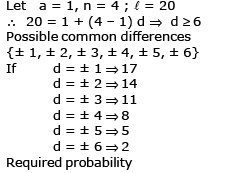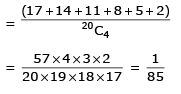QUESTION: 19

Consider 5 independent Bernoulli’s trials each with probability of success p. If the probability of at least one failure is greater than or equal to 31/32,then p lies in the interval :

[AIEEE 2011]

Solution: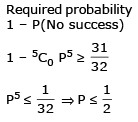QUESTION: 20

If C and D ar e two even ts such th at C ⊂ D and P(D) ≠ 0, th en the cor rect statement among the following is :

[AIEEE 2011]

Solution: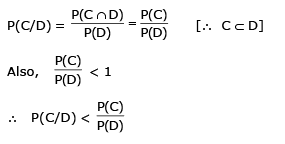QUESTION: 21

Three numbers are chosen at random without replacement from {1, 2, 3, ....8}. The probability that their minimum is 3, given that their maximum is 6, is :

[AIEEE 2012]

Solution:

Let A be the event that minimum is 3 and let B be the event that their maximum is 6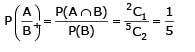QUESTION: 22

A multiple choice examination has 5 questions. Each question has three alternative answers of which exactly one is correct. The probability that a student will get 4 or more correct answers just by guessing is

[JEE -MAIN 2013]

Solution: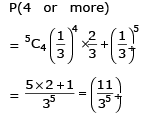QUESTION: 23

If the integers m and n are chosen at random from 1 and 100, then the probability that a number of the form 7m + 7n is divisible by 5 equals

[JEE 99]

Solution:

7m + 7n  divisible by 5
7n[7(m−n)+1]
7(m−n)+1 should be divisible by 5
Power of 7 has last digits revolving in 7,9,3,1.
7(m−n) should have last digit as 9.
7(m−n) = 7(4p+2)
m−n=4p+2
4p+2 ≤ 100
p ≤ 24.5
favorable=25,      total cases=100
P=25/100 = 1/4

QUESTION: 24

The probability that a student passes in Mathematics, Physics and Chemistry are m, p and c respectively.Of these subjects, the student has a 75% chance of passing in at least one, a 50% chance of passing in at least two, and a 40% chance of passing in exactly two, which of the following relations are true ?

Solution: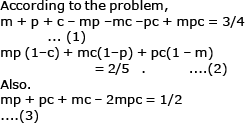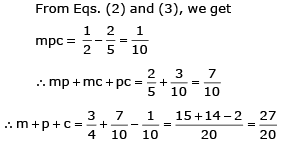QUESTION: 25

Eight players P1, P2, P3, .............P8 play a knockout tournament. It is known that whenever the players Pi and Pj play, the player Pi will win if i < j. Assuming that the players are paired at random in each round, what is the probability that the players P4 reaches the final

Solution: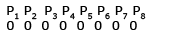Given that if Pi, Pj play with i < j, then Pi will win. For the first round, P4 should be paired with any one from P5 to P8. It can be done in 4C1  ways. Then P4 to be the finalist, at least one player from P5 to P8 should reach in the second round. Therefor, one pair should be from remaining 3 from P\ to P8 in 3C2 Then favourable pairing in first round is 4C1 3C2 3C2. Then in the 2nd round, we have four players.
Favourable ways in 1. Now total possible pairings is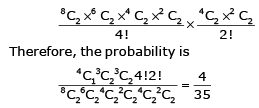*Answer can only contain numeric values
QUESTION: 26

Four cards are drawn from a pack of 52 playing cards. Find the probability of drawing exactly one pair.

[REE 99, 6]

Solution:

There are 13 sets of the same card. We can choose 1 of the 13 in 13C1 = 13 ways. In that set, there are 4 cards. We can select 2 of the 4 in 4C2 =6 ways.The other 2 cards should not form a pair. Hence, we cannot select any card from the set we have selected the first 2 cards. Thus, there are 12 sets left. We need to select 2 sets from the 12 and after that 1 card out of 4 cards from each set. This can be done in 12C2 × 4C1 × 4C1 ways.
Hence, probability = (13 × 4C2 × 12C2 × 4C1 × 4C1)/52C4
= 6336/20825
=0.30

QUESTION: 27

Two cards are drawn at random from a pack of playing cards. Find the probability that one card is a heart and the other is an ace.

[REE 2001 (Mains)]

Solution:

1/26 is the probability of getting a heart or an ace when drawing a single card from a deck of 52 cards.

QUESTION: 28

In a combat, A targets B, and both B and C target A. The probabilities of A, B, C hitting their targets are 2/3, 1/2 and 1/3 respectively. They shoot simultaneously and A is hit. Find the probability that B hits his target whereas C does not.

Solution:

P(A) = A hitting B = ⅔
P(B) = B hitting A = ½
P(C) = C hitting A = ⅓
P(E) = A is hit = P(BUC)
= 1 - P(B’⋂C’)
P(E) = 1 - P(B’)P(C’)
=> 1 - (1 - ½) (1 - ⅓)
=> ⅔
P(B⋂C’/E) = P(B⋂C’)/P(E)
= [P(B) P(C’)]/P(E)
=(½ * ⅔)/(⅔)
= 1/2

QUESTION: 29

Three distinct numbers are selected from first hundred natural numbers. The probability that all the three numbers are divisible by 2 and 3 is

[JEE 2004]

Solution:

If a number is to be divisible by both 2 and 3, it should be divisible by their L.C.M., L.C.M of 2 and 3 is 6. The numbers are 6, 12, 18, ....., 96. The total number is 16.
Hence, the probability is :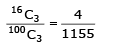QUESTION: 30

A fair dice is thrown until 1 comes, then probability that 1 comes in even number of trials is

[JEE 2005 (Scr.)]

Solution:

In single throw of a dice, probability of getting 1 is 1/6 and probability of not getting 1 is 5/6.
Then, getting 1 in even number of changes is getting 1 in 2nd change or in 4th change or in 6thchange  and so on. Therefore, the required probability is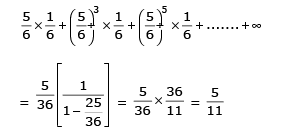QUESTION: 31

There are n urns each containing n + 1 balls such that the ith urn contains i whiteballs and (n + 1 – i) red balls. Let ui be the event of selecting

ith urn, i = 1, 2, 3..........n and W denotes the event of getting a white ball.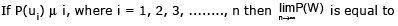[JEE 2006, 5+5+5]

Solution: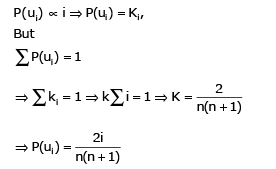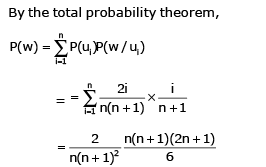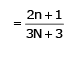QUESTION: 32

There are n urns each containing n + 1 balls such that the ith urn contains i whiteballs and (n + 1 – i) red balls. Let ui be the event of selecting

ith urn, i = 1, 2, 3..........n and W denotes the event of getting a white ball.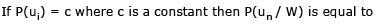Solution: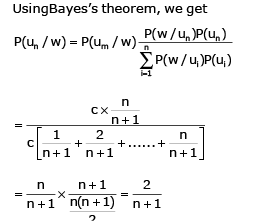QUESTION: 33

If n is even and E denotes the event of choosing even numbered urn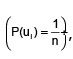then the value of P(W / E), is

Solution: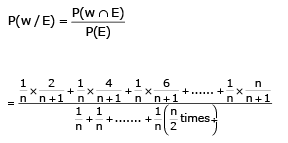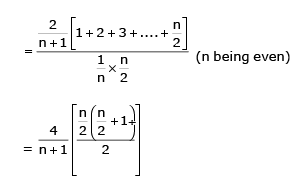QUESTION: 34

An experiment has 10 equally likely outcomes. Let A and B be two non-empty events of the experiment.If A consists of 4 outcomes, the number of outcomes that B must have so that A and B are independent, is

[JEE 2008]

Solution:

Let n(B)and n(A∩B)denote the number outcomes favourable to B and A∩B respectively.
As A and B are independent.
P(A∩B)=P(A)P(B)
⇒ n(A∩B)/10 = 4/10 × n(B)/10
⇒5n(A∩B)=2n(B)
⇒5∣n(B)
⇒nb=5 or 10. [∵1≤nb≤10.]

QUESTION: 35

Consider the system of equations ax + by = 0, cx + dy = 0, where a, b, c, d ∈ {0, 1}

Statement-1 : The probability that the system of equations has a unique solution is 3/8.

Statement-2 : The probability that the system of equations has a solution is 1.

Solution: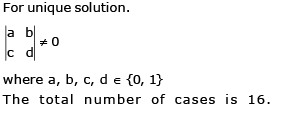Favourable number fo caes is 6 (either ad = 1, bc =0 or ad = 0, bc =1). The probability that system of equation has unique solution is 6/16 = 3/8. Since x = 0 satisfies both the equations, the system has at least one solution.

QUESTION: 36

A fair die is tossed repeatedly until a six is obtained. Let X denA fair die is tossed repeatedly until a six is obtained. Let X denote the number of tosses required. ]The probability that X = 3 equals

[JEE 2009

Solution: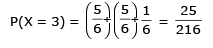QUESTION: 37

A fair die is tossed repeatedly until a six is obtained. Let X denA fair die is tossed repeatedly until a six is obtained. Let X denote the number of tosses required. The probability that X > 3 equals

[JEE 2009]

Solution: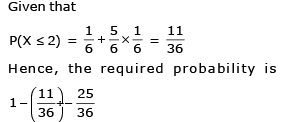QUESTION: 38

A signal which can be green or red with probability 4/5  and 1/5 respectively, is received by station A andthen transmitted to station B. The probability of each station receiving the signal correctly is 3/4, if the signal received at station B is green, then the probability that the original signal was green is

[JEE 2010]

Solution:

Event G = original signal is green

E1 = A recevies the signal correct

E2 = B recevies the signal correct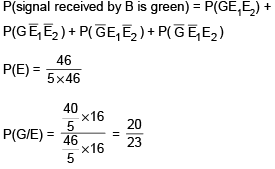QUESTION: 39

Paragraph for Question Nos. 16 to 17

Let U1 and U2 be two urns such that U1 contains 3 white and 2 red balls, and U2 contains only 1 white ball.A fair coin is tossed. If head appears then 1 ball is drawn at random from U1 and put into U2. However, if tail appears then 2 balls are drawn at random from U1 and put into U2. Now 1 ball is drawn at random from U2.

The probability of the drawn ball from U2 being white is

[JEE 2011]

Solution: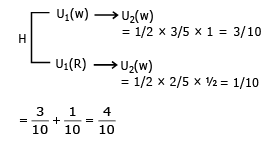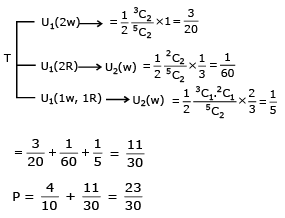QUESTION: 40

Let U1 and U2 be two urns such that U1 contains 3 white and 2 red balls, and U2 contains only 1 white ball.A fair coin is tossed. If head appears then 1 ball is drawn at random from U1 and put into U2. However, if tail appears then 2 balls are drawn at random from U1 and put into U2. Now 1 ball is drawn at random from U2.

Given that the drawn ball from U2 is white, the probability that head appeared on the coin is

Solution: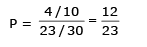QUESTION: 41

Let E and F be two independent events. The probability that exactly one of them occurs is 11/25 and the probability of none of them occurring is 2/25 .If P(T) denotes the probability of occurrence of the event T,, then

[JEE 2011]

Solution: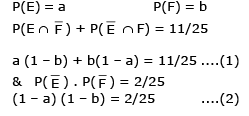*Multiple options can be correct
QUESTION: 42

A ship is fitted with three engines E1, E2 and E3. The engines function independently of each other with respective probabilities 1/2,1/4 and 1/4. For the ship to be operational at least two of its engines must function. Let X denote the event that the ship is operational and let X1, X2 and X3 denote respectively the events that the engines E1 , E2 and E3 are functioning. Which of the following is (are) true ?

[JEE 2012]

Solution: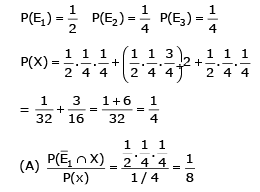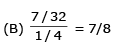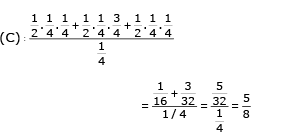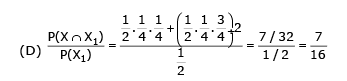QUESTION: 43

Fou r fai r dice D1, D2, D3 and D4, each h avi ng si x faces numbered 1, 2 , 3, 4, 5 and 6, ar e rolled simultaneously. The probability that D4 shows a number appearing on one of D1, D2 and D3 is

[JEE 2012]

Solution:

Four fair dices rolled simultaneously.
Total number of outcomes=6×6×6×6
Suppose, the out come of the last one does not appear in the first three.
The number of such outcomes possible=6×(5)3
(∵D1 can show any of 6 numbers and first three can show any of the remaining 5 numbers)
Hence, probability that D4 does not show a number shown by any of D1,D2 or D3
​=[6×(5)3]/(6)4
So, the required probability =1−[6×(5)3]/(6)4
= 91/(216)

*Multiple options can be correct
QUESTION: 44

Let X and Y be two events such that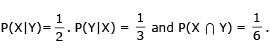Which of the following is (are) correct ?

[JEE 2012]

Solution: